GeeksforGeeks App
Open AppBrowser
Continue

# Decision Making in R Programming – if, if-else, if-else-if ladder, nested if-else, and switch

Decision making is about deciding the order of execution of statements based on certain conditions. In decision making programmer needs to provide some condition which is evaluated by the program, along with it there also provided some statements which are executed if the condition is true and optionally other statements if the condition is evaluated to be false.

The decision making statement in R are as followed:

#### if statement

Keyword if tells compiler that this is a decision control instruction and the condition following the keyword if is always enclosed within a pair of parentheses. If the condition is TRUE the statement gets executed and if condition is FALSE then statement does not get executed.

Syntax:

if(condition is true){

execute this statement

}

Flow Chart: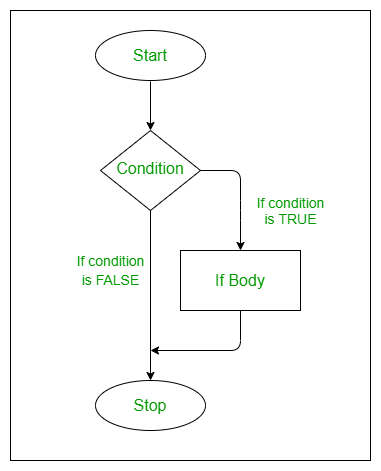Example:

## r

 `# R program to illustrate``# if statement``a <- 76``b <- 67` `# TRUE condition``if``(a > b)``{``    ``c <- a - b``    ``print``(``"condition a > b is TRUE"``)``    ``print``(``paste``(``"Difference between a, b is : "``, c))``}` `# FALSE condition``if``(a < b)``{``    ``c <- a - b``    ``print``(``"condition a < b is TRUE"``)``    ``print``(``paste``(``"Difference between a, b is : "``, c))``}`

Output:

``` "condition a > b is TRUE"
 "Difference between a, b is :  9"```

#### if-else statement

If-else, provides us with an optional else block which gets executed if the condition for if block is false.  If the condition provided to if block is true then the statement within the if block gets executed, else the statement within the else block gets executed.

Syntax:

if(condition is true) {

execute this statement

} else {

execute this statement

}

Flow Chart: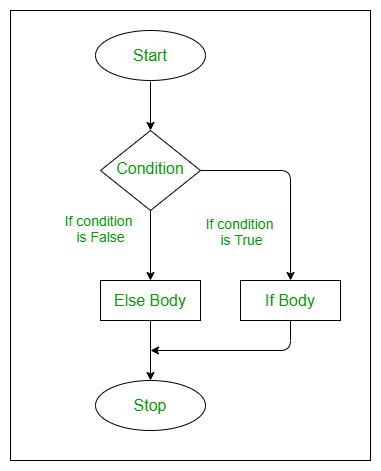Example :

## r

 `# R if-else statement Example``a <- 67``b <- 76`  `# This example will execute else block``if``(a > b)``{``    ``c <- a - b``    ``print``(``"condition a > b is TRUE"``)``    ``print``(``paste``(``"Difference between a, b is : "``, c))``} ``else``{``    ``c <- a - b``    ``print``(``"condition a > b is FALSE"``)``    ``print``(``paste``(``"Difference between a, b is : "``, c))``}`

Output:

``` "condition a > b is FALSE"
 "Difference between a, b is :  -9"```

It is similar to if-else statement, here the only difference is that an if statement is attached to else. If the condition provided to if block is true then the statement within the if block gets executed, else-if the another condition provided is checked and if true then  the statement within the block gets executed.

Syntax:

if(condition 1 is true) {

execute this statement

} else if(condition 2 is true) {

execute this statement

} else {

execute this statement

}

Flow Chart: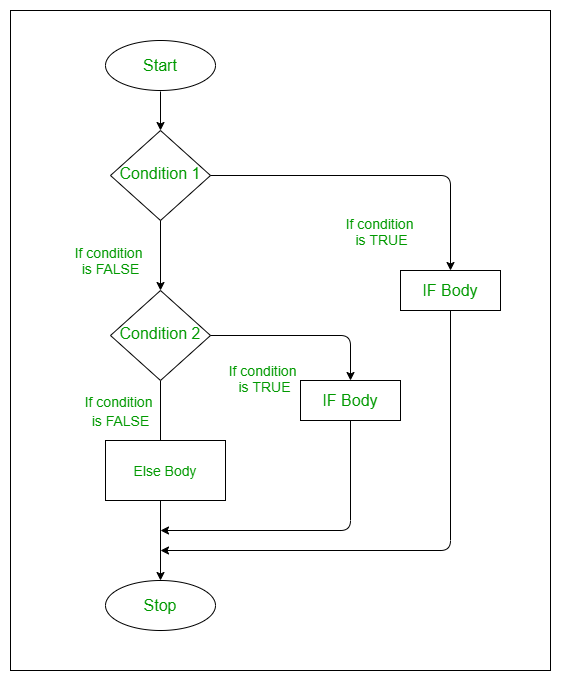Example :

## r

 `# R if-else-if ladder Example``a <- 67``b <- 76``c <- 99`  `if``(a > b && b > c)``{``    ``print``(``"condition a > b > c is TRUE"``)``} ``else` `if``(a < b && b > c)``{``    ``print``(``"condition a < b > c is TRUE"``)``} ``else` `if``(a < b && b < c)``{``    ``print``(``"condition a < b < c  is TRUE"``)``}`

Output:

` "condition a < b < c  is TRUE"`

#### Nested if-else statement

When we have an if-else block as an statement within an if block or optionally within an else block, then it is called as nested if else statement. When an if condition is true then following child if condition is validated and if the condition is wrong else statement is executed, this happens within parent if condition. If parent if condition is false then else block is executed with also may contain child if else statement.

Syntax:

if(parent condition is true) {

if( child condition 1 is true) {

execute this statement

} else {

execute this statement

}

} else {

if(child condition 2 is true) {

execute this statement

} else {

execute this statement

}

}

Flow Chart: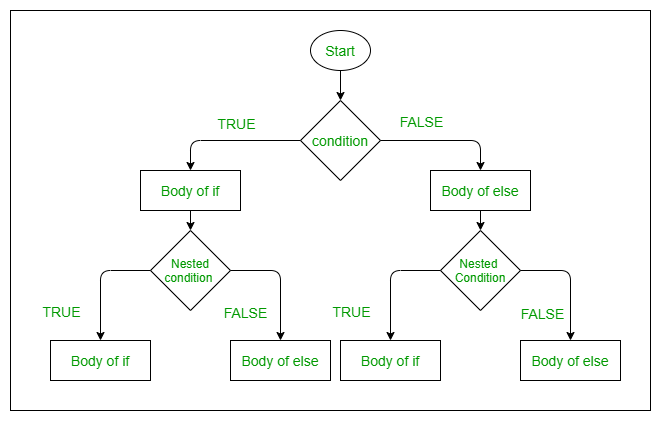Example:

## r

 `# R Nested if else statement Example``a <- 10``b <- 11`  `if``(a == 10)``{``    ``if``(b == 10)``    ``{``        ``print``(``"a:10 b:10"``)   ``    ``} ``else``    ``{``        ``print``(``"a:10 b:11"``)    ``    ``}``} ``else``{``    ``if``(a == 11)``    ``{``        ``print``(``"a:11 b:10"``)``    ``} ``else``    ``{``        ``print``(``"a:11 b:11"``)``    ``}``}`

Output:

` "a:10 b:11"`

#### switch statement

In this switch function expression is matched to list of cases. If a match is found then it prints that case’s value. No default case is available here. If no case is matched it outputs NULL as shown in example.

Syntax:

switch (expression, case1, case2, case3,…,case n )

Flow Chart :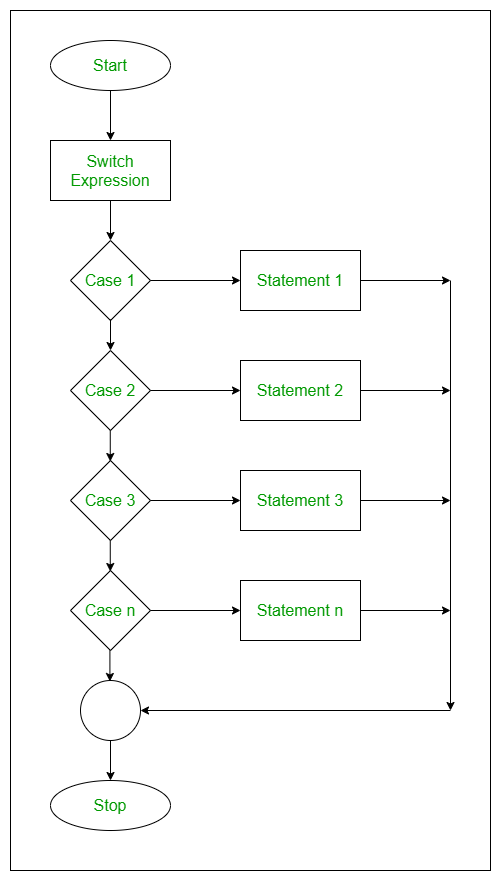Example:

## r

 `# R switch statement example` `# Expression in terms of the index value``x <- ``switch``(``    ``2,           ``# Expression``    ``"Geeks1"``,    ``# case 1``    ``"for"``,       ``# case 2``    ``"Geeks2"`     `# case 3``)``print``(x)` `# Expression in terms of the string value``y <- ``switch``(``    ``"GfG3"``,              ``# Expression``    ``"GfG0"``=``"Geeks1"``,     ``# case 1``    ``"GfG1"``=``"for"``,        ``# case 2``    ``"GfG3"``=``"Geeks2"`      `# case 3``)``print``(y)` `z <- ``switch``(``    ``"GfG"``,               ``# Expression``    ``"GfG0"``=``"Geeks1"``,     ``# case 1``    ``"GfG1"``=``"for"``,        ``# case 2``    ``"GfG3"``=``"Geeks2"`      `# case 3``)``print``(z)``   ``print``(z)`

Output:

``` "for"
 "Geeks2"
NULL```

My Personal Notes arrow_drop_up